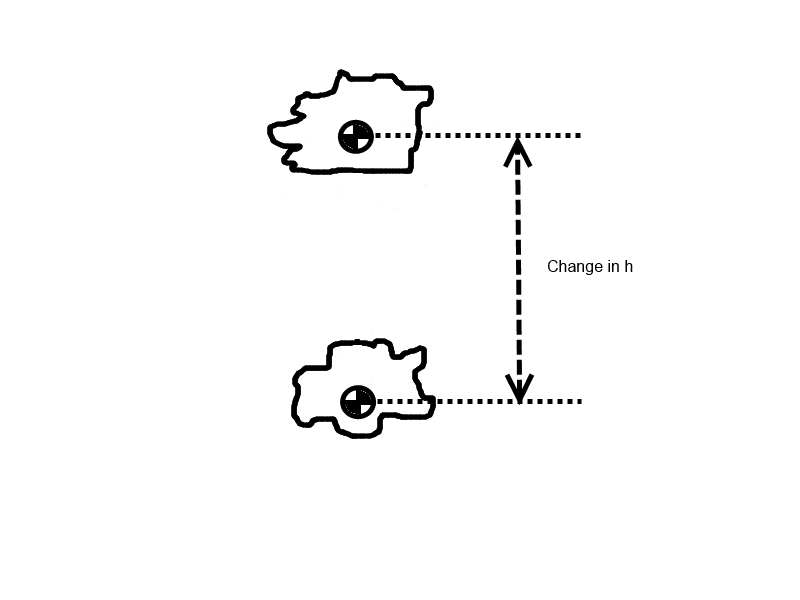# Using center of mass for loop de loop

• B
A quick question, I remember my professor said to use the center of mass for solving the classic loop de loop problem. For example, he wanted us to use the distance from the surface of the loop to the center of the object (instead of just from the loop to the surface of the block) for the height in PE = mgh. Thinking back on this, it seems pretty weird that this was done. What do you guys think?

Doc Al
Mentor
Often the size of the object is assumed negligible compared to other distances in the problem. But when you calculate changes in the gravitational PE of an object, it makes sense to use changes in the center of mass position.

Often the size of the object is assumed negligible compared to other distances in the problem. But when you calculate changes in the gravitational PE of an object, it makes sense to use changes in the center of mass position.
So lets say the mass is at rest at the highest position, and is just about to fall. So its potential energy would be say mg(51) instead of mg(50)? And at the zero reference point (ground), its potential energy is still mg(1)?

Doc Al
Mentor
So lets say the mass is at rest at the highest position, and is just about to fall. So its potential energy would be say mg(51) instead of mg(50)? And at the zero reference point (ground), its potential energy is still mg(1)?
If you measure PE from ground level, when the object is resting on the ground its PE = mgd (say). Then when it's at the highest point, resting on top of the track at height h, its PE would be mg(h+d). For some things it doesn't matter, since the change in PE will still be mgh.

It depends on exactly what you are trying to calculate.

If you measure PE from ground level, when the object is resting on the ground its PE = mgd (say). Then when it's at the highest point, resting on top of the track at height h, its PE would be mg(h+d). For some things it doesn't matter, since the change in PE will still be mgh.

It depends on exactly what you are trying to calculate.
I see, I just find it weird that its potential energy would be non-zero at ground level. When would one want to use the center of mass to calculate PE?

Doc Al
Mentor
I see, I just find it weird that its potential energy would be non-zero at ground level.
Realize that the zero level is arbitrary. Only changes in gravitational PE have meaning.

When would one want to use the center of mass to calculate PE?
Depends on how accurate you want to be and what you are asked to calculate.

Realize that the zero level is arbitrary. Only changes in gravitational PE have meaning.

Depends on how accurate you want to be and what you are asked to calculate.
In the classic problem, where it asks to calculate the energy needed to get pass the loop, is this required? I'm having trouble thinking up with situations where it would want to be done. What is the size difference to make this matter?

Doc Al
Mentor
In the classic problem, where it asks to calculate the energy needed to get pass the loop, is this required?
Think about it. What's the PE initially? What's the PE of the object when it passes the top of the loop? (Note that the object is now below the track.) Since centripetal acceleration is involved, what radius will you use?

I'm having trouble thinking up with situations where it would want to be done. What is the size difference to make this matter?
It depends how accurate you want to be. Many versions of the problem don't even give the dimensions of the object, so you are expected to ignore it.

Think about it. What's the PE initially? What's the PE of the object when it passes the top of the loop? (Note that the object is now below the track.) Since centripetal acceleration is involved, what radius will you use?
For without accounting for the center of mass:
y = 2r + 0.5r

For accounting for the center of mass,
y = 2r + 0.5r - 2x

(y is the minimum height needed to pass the loop, r is radius of the loop, and x is the distance to the center of mass)
So it would matter right?

Doc Al
Mentor
For without accounting for the center of mass:
y = 2r + 0.5r
OK.

For accounting for the center of mass,
y = 2r + 0.5r - 2x
How did you arrive at this?

So it would matter right?
Sure. As to how much it matters, that depends on how x compares to r.

How did you arrive at this?
mg(y+x) = mg(2r-x) + 0.5mrg
y + x = 2r - x + 0.5r

Doc Al
Mentor
mg(y+x) = mg(2r-x) + 0.5mrg
y + x = 2r - x + 0.5r
Check that 0.5mrg term. Recall my comment about what radius to use.

•Sho Kano
Check that 0.5mrg term. Recall my comment about what radius to use.
0.5mrg should be 0.5m(r-x)g?

Doc Al
Mentor
0.5mrg should be 0.5m(r-x)g?
Right.

CWatters
Homework Helper
Gold Member
Perhaps it helps to think about the case where the object is an irregular shape or even flexible with no obvious "surface" to measure from. Something like a rain cloud....Doc Al
Mentor
Perhaps it helps to think about the case where the object is an irregular shape or even flexible with no obvious "surface" to measure from. Something like a rain cloud....
That will certainly make things more interesting!

I, of course, was speaking of the simpler case of a rigid object (a cart, for example) with a well-defined center of mass.

CWatters